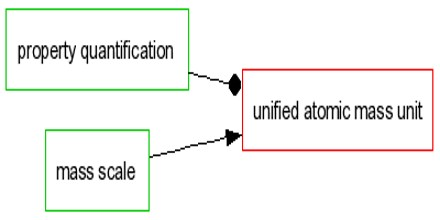Unified Atomic Mass Unit

Definition

The mass of the unified atomic mass unit is determined experimentally. It is a unit of atomic and molecular mass. By definition it is one twelfth of the mass of an unbound carbon-12 (12C) atom, at rest and in its ground state.

The relationship of the unified atomic mass unit to the macroscopic SI base unit of mass, the kilogram, is given by Avogadro’s number NA. By the definition of Avogadro’s number, the mass of NA carbon-12 atoms, at rest and in their ground state, is 12 gram (= 12×10−3 kg). The latest value of NA is the same numerical value as the Avagadro constant, the number of “elementary entities” in a mole of substance:

NA = 6.022 141 29(27) × 1023 / mol,

Where the symbol mol represents the mole, which can be defined for any substance as a sample containing the same number of “elementary entities” (atoms or molecules, for example) as there are atoms of 12C in 0.012 kg of carbon-12.The amu without the “unified” prefix is technically an obsolete unit based on oxygen, which was replaced in 1961. However, many sources still use the term “amu” but now define it in the same way as u (i.e., based on carbon-12). In this sense, most uses of the terms “atomic mass units” and “amu” today actually refer to unified atomic mass unit.

The chemistry amu, based on the relative atomic mass (atomic weight) of natural oxygen, including the heavy naturally-occurring isotopes 17O and 18O, which was about 1.000282 as massive as the physics amu, based on pure isotopic 16O. The choice of carbon-12 was made to minimise further divergence with prior literature. The new and current unit was referred to as the “unified atomic mass unit” u. and given a new symbol, “u,” which replaced the now deprecated “amu” that had been connected to the old oxygen-based system. The Dalton (Da) is another name for the unified atomic mass unit.Unified Atomic Mass Unit Relationship to SI

The definition of the mole, an SI base unit, was accepted by the CGPM in 1971 as:

• The mole is the amount of substance of a system which contains as many elementary entities as there are atoms in 0.012 kilogram of carbon-12; its symbol is “mol”.
• When the mole is used, the elementary entities must be specified and may be atoms, molecules, ions, electrons, other particles, or specified groups of such particles.

The definition of the mole also determines the value of the universal constant that relates the number of entities to amount of substance for any sample. This constant is called the Avogadro constant, symbol NA or L, and has the value 6.022140857(74) ×1023 mol−1 (entities per mole).

Given that the unified atomic mass unit is one twelfth the mass of one atom of carbon-12, meaning the mass of such an atom is 12 u, it follows that there are NA atoms of carbon-12 in 0.012 kg of carbon-12. This can be expressed mathematically as

NA (12 u) = 0.012 kg/mol, or

NA u = 0.001 kg/molConfusions about Unified Atomic Mass Unit

In the system of atomic units, the “atomic unit of mass” is the mass of the electron me. But further confusion prevails:

In the literature one still finds the obsolete unit amu (atomic mass unit). This antiquated usage is deplorable, not only because the amu is not a unit accepted for use with the SI, but also because two different standard masses are denoted by amu. There is the physicist’s amu (= 1/1.000 317 9 u) and there is the chemist’s amu (= 1/1.000 043 u). Because chemists and physicists now use the same atomic mass unit, today it is referred to as unified. It is a non-SI unit accepted for use with the SI, whose value in SI units is obtained experimentally.